# XDCR Data-Type Conversion

+
XDCR filtering expressions are supported by data-type conversion and collation.

## Understanding Data-Type Conversion

XDCR filtering expressions allow case-sensitive matches, comparisons, logical and other operations to be performed on values within documents. When values referenced by a filtering expression have different data types, either conversion or collation is performed:

• Conversion means that one or both of the values is converted from its own data type to a different one, so that the operation can be performed.

• Collation means that conversion is not possible; and that therefore, the operation will be resolved by value-comparison according to a set of rules.

All conversion performed by XDCR is implicit, meaning that it is performed automatically within the system. No explicit conversion (that is, data-type conversion selected and imposed by the administrator) is supported.

## Supported Data Types

The data types supported by XDCR Filtering are listed below, in descending order of precedence — the lowest precedence-value (and earliest position) therefore indicating the highest precedence.

 Precedence Data Type 0 Invalid 1 Missing 2 Null 3 Boolean 4 Numeric 5 String 6 Time 7 Array 8 Object 9 Binary

When an expression entails values of the same data type, no conversion is required. When the data types differ, and conversion or collation is therefore required, data types take precedence in accordance with their closeness to the top of the list. For example, if an operation refers to two values, one of which is Invalid and the other of which is Boolean, the Invalid value determines the result. As another example, if an operation refers to two values, one of which is Boolean and the other Numeric, the Numeric value is if possible converted to Boolean, and the operation performed.

The data types listed above, and their order of precedence, can be compared with those used by N1QL — which are similar, but not identical.

See XDCR Filtering Expressions, for information on how comparisons can be performed, using the data types listed above.

## Numeric Data-Type Internal Conversions

A value of the Numeric data type (listed above, in Supported Data Types) always further corresponds to one of three internal numeric data types; which are unsigned integer, integer, and float. When two Numeric data-type values are referenced in a filtering expression, comparisons (e.g. equality, inequality) and operations (e.g. addition, subtraction) are performed as indicated in the following table.

Type 1 Type 2 Comparison Operation

`Uint`

`Uint`

The comparison is performed.

The operation is performed. If the operation results in a negative value, both values are converted to `Int`, and the operation is repeated; unless one or both conversions to `Int` would result in overflow, in which case `InvalidValue` is returned.

`Uint`

`Int`

If `Int` is negative, `Uint` is converted to `Int`, and the comparison is performed; unless the conversion to `Int` would result in overflow, in which case `InvalidValue` is returned.

If `Int` is positive, `Int` is converted to `Uint`, and the comparison is performed.

The operation is performed. If the result is an `Int`, the `Int` is returned. If the result is a valid `Uint`, the `Uint` is returned. If the result would be non-valid as a `Uint` (due to the result’s being negative), the result is converted to `Int`, and is returned as such; unless the conversion to `Int` would result in overflow, in which case, `InvalidValue` is returned.

`Uint`

`Float`

`Uint` is converted to `Float`, and the comparison is performed.

`Uint` is converted to `Float`, and the operation is performed.

`Int`

`Float`

`Int` is converted to `Float`, and the comparison is performed.

`Int` is converted to `Float`, and the operation is performed.

`Float`

`Float`

The comparison is performed.

The operation is performed.

`Int`

`Int`

The comparison is performed.

The operation is performed.

## Implicit Conversion versus Collation Comparison

Implicit conversion occurs when Couchbase Server converts a value from its existing data type to another, so that a comparison or operation can be performed on values of the same data type. Implicit conversion is only sometimes possible: therefore, when an existing data type cannot be implicitly converted into another, collation comparison is used instead.

Collation comparison works as follows:

• Equality and inequality. Checking for equality between different data types returns `false`. Therefore, if `var1` and `var2` represent different data types, the expression `var1 == var2` returns `false`.

Checking for inequality between different data types returns `true`. Therefore, if `var1` and `var2` represent different data types, the expression `var1 != var2` returns `true`.

• Regular expression matches. Checking for a regular-expression match between different data types returns `false`. Therefore, if `var1` and `var2` represent different data types, the expression `REGEXP_CONTAINS(var1, var2)` returns `false`.

Checking for a regular-expression non-match between different data types returns `true`. Therefore, if `var1` and `var2` represent different data types, the expression `NOT REGEXP_CONTAINS(var1, var2)` returns `true`.

• Magnitude comparison between different data types is resolved in accordance with the respective positions of the data types in the list of Supported Data Types). This list presents the supported data types in order of precedence: note that precedence and magnitude are inversely proportional; and that therefore, the data type at the bottom of the list (Binary) has the least precedence and greatest magnitude; while the data type at the top (Invalid) has the greatest precedence and least magnitude.

Consequently, if the data type of `var1` is below that of `var2` in the list, the expression `var1 > var2` returns `true`; and the expression `var1 < var2` returns `false`.

## Supported and Unsupported Type Conversions

The following diagram lists supported and unsupported type conversions. Conversion-support is indicated by the following:

•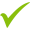Conversion can be performed.

•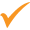Conversion can be performed under certain conditions.

•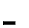Conversion is not required.

•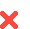Conversion cannot be performed.

Each cell in the diagram bears one or more integers: these correspond to explanatory annotations that are listed further below.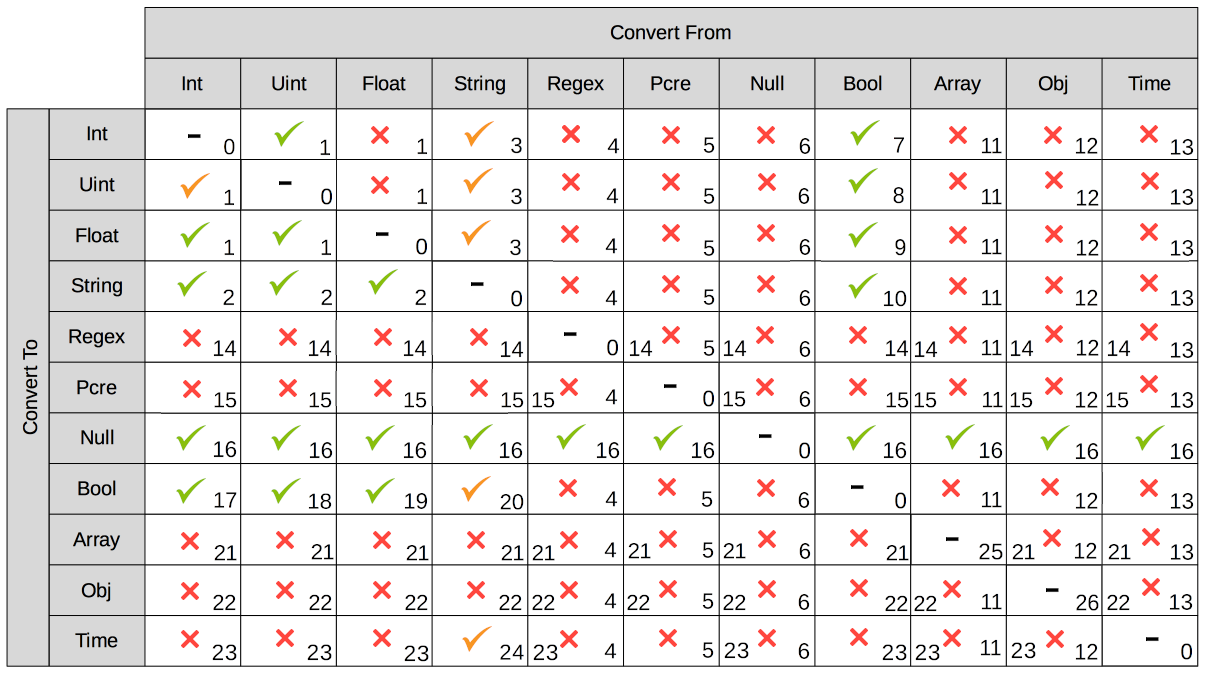These conversion-support options are described in the following table, each row of which starts with an integer that corresponds to an annotation in the diagram above. Notes on comparison-procedures are also provided.

# From To Validity Comparison

0

`<Any-Except-Boolean-and-Object>`

`<Same-Type>`No conversion need be performed.

Standard comparison for the type.

1

`Numeric`

`Numeric`Valid, possibly valid, or invalid. See Numeric Data-Type Internal Conversions, above.

See Numeric Data-Type Internal Conversions, above, for details on comparison.

2

`Numeric`

`String`Valid for `Int`, `Uint`, and `Float`. In each case, `Numeric` is converted to `String`.

Standard string-comparison is performed.

3

`String`

`Numeric`Valid if `String` can be converted to `Int`; otherwise valid if `String` can be converted to `Float`; otherwise invalid.

Standard numeric-comparison is performed, if possible. Otherwise, collation comparison is performed.

4

`Regex`

`<Any-Except-Regex-and-Null>`Invalid. No conversion can occur, except to `Null`.

Collation comparison is performed, except for `Regex` and `Null`.

5

`Pcre`

`<Any-Except-Pcre-and-Null>`Invalid. No conversion can occur, except to `Null`.

Collation comparison is performed, except for `Pcre` and `Null`.

6

`Null`

`<Any-Except-Null>`Invalid. No conversion can occur.

Standard comparison for the type.

7

`Boolean`

`Int`Valid. The `Boolean` values `true` and `false` are converted to the `Int` values `1` and `0` respectively.

Standard numeric-comparison is performed.

8

`Boolean`

`Uint`Valid. The `Boolean` values `true` and `false` are converted to the `Uint` values `1` and `0` respectively.

Standard numeric-comparison is performed.

9

`Boolean`

`Float`Valid. The `Boolean` values `true` and `false` are converted to the `Float` values `1.0` and `0.0` respectively.

Standard numeric-comparison is performed.

10

`Boolean`

`String`Valid. A `Boolean` can be converted to a `String` whose value is either `"true"` or `"false"`

The string-comparison `"true" > "false"` returns `true`.

11

`Array`

`<Any-Except-Array-and-Null>`Invalid.

Collation comparison is performed for all except `Array` and `Null`.

12

`Object`

`<Any-Except-Object-and-Null>`Invalid.

Collation comparison is performed for all except `Object` and `Null`.

13

`Time`

`<Any-Except-Time-and-Null>`Invalid.

Collation comparison is performed for all except `Time` and `Null`.

14

`<Any-Except-Regex>`

`Regex`Invalid.

Collation comparison is performed for all except `Regex`.

15

`<Any-Except-Pcre>`

`Pcre`Invalid.

Collation comparison is performed for all except `Pcre`.

16

`<Any-Except-Null>`

`Null`Valid. `<Any>` is converted a `non-Null` value, for comparison with `Null`.

The comparison `non-Null > Null` returns `true`.

17

`Int`

`Boolean`Valid for all `Int` values. The `Int` value `0` is converted to the `Boolean` value `false`; all other `Int` values are converted to the `Boolean` value `true`.

The boolean-comparison `true > false` returns `true`.

18

`Uint`

`Boolean`Valid for all `Uint` values. The `Uint` value `0` is converted to the `Boolean` value `false`; all other `Uint` values are converted to the `Boolean` value `true`.

The boolean-comparison `true > false` returns `true`.

19

`Float`

`Boolean`Valid for all `Float` values. The `Float` value `0.0` is converted to the `Boolean` value `false`; all other `Float` values are converted to the `Boolean` value `true`.

The boolean-comparison `true > false` returns `true`.

20

`String`

`Boolean`Valid if `String` is case-insensitive `"true"` or `"false"`; in which case `String` is converted to its `Boolean` equivalent.

The boolean-comparison `true > false` returns `true`.

21

`<Any-Except-Array>`

`Array`Invalid.

Collation comparison is performed.

22

`<Any-Except-Object>`

`Object`Invalid.

Collation comparison is performed.

23

`<Any-Except-Time-and-String`

`Time`Invalid.

Collation comparison is performed.

24

`String`

`Time`Valid if `String` can be parsed as a parameter to the `DATE` function.

Standard comparison is performed if valid; otherwise, collation comparison is performed.

25

`Array`

`Array`No conversion need be performed.

See the Note for Row 25, below.

26

`Object`

`Object`No conversion need be performed.

See the Note for Row 26, below.

### Note for Row 25

Comparison is performed along the following lines: the specified expression is first applied to the arrays themselves, based on the respective array-lengths. For example, if the expression is `arg_1 > arg_2`, this gets applied as `Array_1 > Array_2`; and if the length of `Array_1` is indeed greater than the length of `Array_2`, the condition is considered to have been met; whereby `true` is returned, and the comparison-process ends.

In cases where the condition specified by the expression is one of inequality (i.e., `>`, `<`, `!=`), the array-lengths are not equal, and the specified condition is not met (for example, where the expression is `arg_1 > arg_2`, and the length of `Array_1` is less than that of `Array_2`), `false` is returned, and the comparison-process ends.

In cases where the condition specified by the expression is one of inequality, and the condition is not met due to the array-lengths being equal, the comparison-process continues as follows: in sequence, pairs of correspondingly positioned objects from the arrays are compared, until the specified requirement is met, or is finally determined not to have been met. For example, if the expression is `arg_1 > arg_2`, this gets applied as `Array_1 > Array_2`, `Array_1 > Array_2`, and so forth.

### Note for Row 26

Comparison is performed along the following lines: the specified expression is first applied to the objects in terms of their respective lengths. For example, if the expression is `arg_1 > arg_2`, this gets applied as `Object_1 > Object_2`; and if the length of `Object_1` is indeed greater than the length of `Object_2`, the requirement is considered to have been met; whereby `true` is returned, and the comparison-process ends.

In cases where the condition specified by the expression is one of inequality (i.e., `>`, `<`, `!=`), the object-lengths are not equal, and the specified condition is not met (for example, where the expression is `arg_1 > arg_2`, and the length of `Object_1` is less than that of `Object_2`), `false` is returned, and the comparison-process ends.

In cases where the condition specified by the expression is one of inequality, and the condition is not met due to the object-lengths being equal, the comparison-process continues as follows: in sequence, pairs of correspondingly positioned data-bytes from the objects are compared, until the specified requirement is met, or is finally determined not to have been met. For example, if the expression is `arg_1 > arg_2`, this gets applied as `Object_1 > Object_2`, `Object_1 > Object_2`, and so forth.

## Implicit Conversion Modes

Each filter expression requires implicit conversion to be applied to one of the following combinations:

• A Constant and a Variable. The filter expression contains a user-specified constant and a variable, which are to be compared. Couchbase Server determines the data type of the constant, and attempts to apply this data type to the value of the variable.

• Two Variables. The filter expression specifies two variables, which are to be compared. Couchbase Server determines the data type of the variables from the corresponding values in the JSON document to which filtering is currently being applied.

These modes are described in the subsections below.

### Implicit Conversion of Constant and Variable.

When a constant and a variable are to be compared, Couchbase Server determines the data type of the constant, and attempts to apply this also to the value of the variable.

#### Data-Type Conversion of User-Specified Constants

When the user explicitly enters a constant into a filter expression, the data type for the constant is evaluated by Couchbase Server, as part of its process for tokenizing the expression (that is, parsing the expression into identifiable lexical components).

The correspondences between token formats and duly assigned data types is described in the following table.

Token Format Assigned Data Type Example

Any character-sequence enclosed either by double quotes (`""`) or by single quotes (`''`).

`String`

`variable == "a string"`

`Variable == 'another string'`

Any numeric values without precision delimiter or mantissa.

`Int`

`variable > 1234`

`variable < -2345`

Any number representing a valid `golang` float, optionally with precision delimiter and/or mantissa.

`Float`

`variable >= 1.2343e+25`

Any of the following, specified without enclosing punctuation (such as commas or inverted commas): `true`, `TRUE`, `false`, `FALSE`.

`Boolean`

`variable == true`

`variable != FALSE`

Either of the keyword-phrases `IS NULL` and `IS NOT NULL`.

`null`

`variable IS NULL`

`variable IS NOT NULL`

Valid hard-coded strings wrapped by the `DATE()` function.

`date`

`variable < DATE("2018-10-17T00:01:02Z")`

##### Mathematical Data Types

Mathematical expressions may be entered as constants.

Division

When an expression includes a division operation, the result of which is intended to be a decimal number, the operands themselves must be specified as (or, if they are variables, allowed to be implicitly cast to) decimals.

For example, if `A == 4` returns `true`, then `1 / A == 0.25` returns `false`; because the expression `1 / A` casts `A` implicitly to `Int`, and duly returns an `Int`. Thus, `Int(1 / 4) == 0` returns `true`.

On the other hand, `1.0 / A == 0.25` returns `true`; because the expression `1.0 / A` casts `A` implicitly to `Float`, and duly returns a `Float`. Thus, `1.0 / 4.0 == 0.25` returns `true`.

Not-a-Number Values

`NaN` (Not-a-Number) float-values are considered less than any other real number.

Two `NaNs` do not yield equality. Note, however, that the operators `<=` and `=>` return `true`: this differs from the `golang` standard (according to which these operators return `false`).

#### Data-Type Conversion of Variable-Values, for Comparison with Constants

When an expression includes both a constant and a variable, Implicit Conversion to Constant Data-Type is performed on the constant. Then, the data-type derived from evaluation of the constant is assigned, attemptedly, to the value represented by the variable.

For example, given the expression `variable > 4.5`, the constant `4.5` is determined to be a `Float`. The value of `variable` is then examined; and if determined not itself to be a `Float`, is attemptedly converted to `Float`.

If conversion of the variable’s value into the data type specified by the user is not possible, collation comparison is performed. For example, given the expression `variable != true`, if the value of `variable` is not `Boolean`, collation comparison is performed, and `true` is returned, due to the data types' being different.

If the data-type of the user-specified constant is `Numeric`, and the value of the variable is also `Numeric`, the appropriate numeric data-type internal conversion is performed (see above).

If the value is `NaN`, the value is converted to the `Invalid` data-type, and collation comparison is performed. For example, given the expression `ASIN(variable) > 0`, if the value of `variable` is `93`, then `ASIN(93)` results in a `NaN` value, which is then duly converted to `Invalid`. Collation comparison is then performed, and returns that the `NaN` is smaller than the `Int`.

Alternatively, given the expression `ASIN(variable) != 0`, collation comparison returns `true`, due to the comparison’s being made between different data types (`Invalid` and `Int`).

An explanation of comparisons with `NaN` values is provided in Not-a-Number Values, above.

### Implicit Conversion between Variables

When an expression consists entirely of variables (for example, `variable != otherVariable`), Couchbase Server retrieves the corresponding values from the JSON document to which filtering is currently being applied, and performs conversions on the values.

The result of conversion may vary for each variable, document by document, based on changes encountered in JSON definitions.

Couchbase Server performs conversion differently, according to whether both values are determined to be `Numeric`.

#### Numeric Comparison

If the values of both variables are determined to be `Numeric`, the appropriate numerical comparison from those described in Data Type Conversion of User-Specified Constants is made.

#### Non-Numeric Comparison

If the values for the variables are not both numeric, both require non-numeric conversion. Conversion is performed based on the following sequence:

1. Whether the perceived types support comparison. See the diagram in Supported and Unsupported Type Conversions for information.

2. Whether, if the perceived types do support comparison, a successful attempt can be made to convert the less restrictive data type to the more restricted. See the table in Supported Data Types, for information.

3. Whether, if the less restrictive data type cannot be converted to the more restricted, the more restricted can be converted to the less.

If conversion cannot be achieved by the above sequence, collation comparison is performed.

For example, given the expression `variable1 > variable2`, where `variable1` is the `Boolean` value `true`, and `variable2` is the `String` value `"test"`:

1. The perceived types are checked as to whether they support direct comparison. They do not.

2. The `String` value `test` is attemptedly cast to a `Boolean`: which fails.

3. The `Boolean` value `true` is attemptedly cast to a `String`: which succeeds — the new `String` value being `"true"`.

A comparison between the strings is now performed, with the result being `true`.

Alternatively, given the same expression, where `variable1` is now the `String` value `"test"`, and `variable2` is a JSON array; conversion cannot be achieved by the sequence. Therefore, collation comparison is performed; the result of which is `false`, because `Array` is of lower precedence (and therefore, higher magnitude) than `String` — see Supported Data Types for the precedence-list of data types.

Output